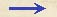Discorsi Propositions 2/28-pr-11 Discorsi Proposition2/28-pr-11{249} PROBLEMA XI, PROPOSITIO XXVIII. {249} PROBLEM XI, PROPOSITION XXVIIITangat horizontalis linea AG circulum, et a contactu sit diameter AB, et duae chordae utcunque AEB: determinanda sit ratio temporis casus per AB ad tempus descensus per ambas AEB. Let AG be any horizontal line touching a circle; let AB be the diameter passing through the point of contact; and let AE and EB represent any two chords.The problem is to determine what ratio the time of fall through AB bears to the time of descent over both AE and EB. Extendatur BE usque ad tangentem in G, et angulus BAE bifariam secetur, ducta AF: dico, tempus per AB ad tempus per AEB esse ut AE ad AEF. Cum enim angulus FAB aequalis sit angulo FAE, angulus vero EAG angulo ABF, erit totus {10} GAF duobus FAB, ABF aequalis; quibus aequatur quoque angulus GFA; ergo linea GF ipsi GA est aequalis: et quia rectangulum BGE aequatur quadrato GA, erit quoque aequale quadrato GF, et tres lineae BG, GF, GE proportionales. Quod si ponatur, AE esse tempus per AE, erit GE tempus per GE, et GF tempus per totam GB, et EF tempus per EB, post descensum ex G seu ex A per AE: tempus igitur per AE, seu per AB, ad tempus per AEB est ut AE ad AEF: quod erat determinandum. {20} Aliter brevius. Secetur GF aequalis GA; constat, GF esse mediam proportionalem inter BG, GE. Reliqua ut supra. Extend BE till it meets the tangent at G, and draw AF so as to bisect the angle BAE. Then, I say, the time through AB is to the sum of the times along AE and EB as the length AE is to the sum of the lengths AE and EF. For since the angle FAB is equal to the angle FAE, while the angle EAG is equal to the angle ABF it follows that the entire angle GAF is equal to the sum of the angles FAB and ABF. But the angle GFA is also equal to the sum of these two angles. Hence the length GF is equal to the length GA; and since the rectangle BG.GE is equal to the square of GA, it will also be equal to the square of GF, or BG.GF = GF:GE. If now we agree to represent the time of descent along AE by the length AE, (Condition 2/03-th-03-cor) then the length GE will represent the time of descent along GE, (Condition 2/02-th-02-cor2) while GF will stand for the time of descent through the entire distance GB; (Condition 2/11-th-11) so also EF will denote the time through EB after fall from G or from A along AE. Consequently the time along AE, (Condition 2/06-th-06) or AB, is to the time along AE and EB as the length AE is to AE+EF. Q. E. D.A shorter method is to lay off GF equal to GA, thus making GF a mean proportional between BG and GE.The rest of the proof is as above.Discorsi Propositions 2/28-pr-11 Discorsi Proposition2/28-pr-11